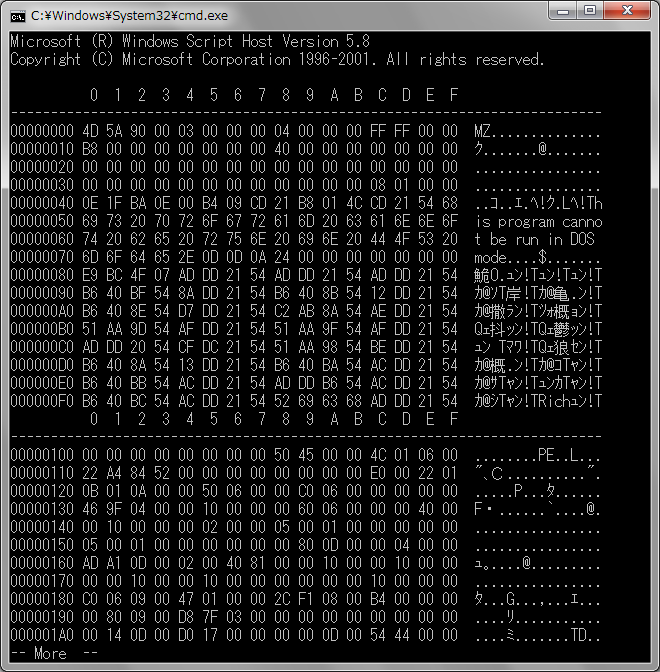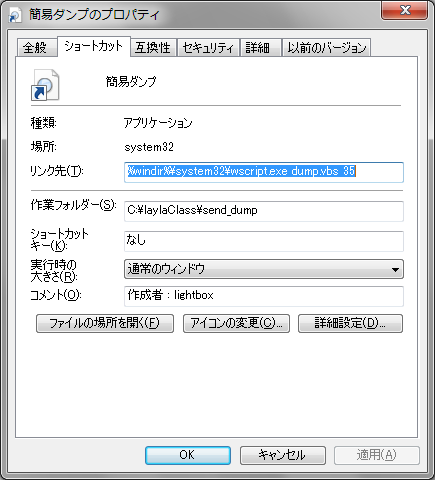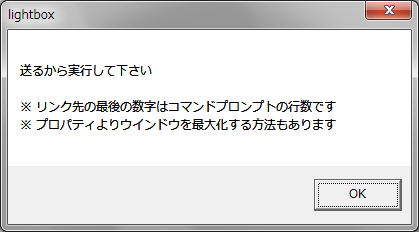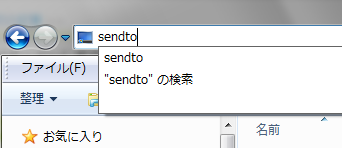ＳＱＬの窓

## 2019年02月15日

### 「送る」からファイルのダンプ

```※コマンドプロンプトの操作
Q : 終了します。
スペースキー : 次ページを表示します。
Enterキー : 次の行を表示します

SendTo フォルダにショートカットを作成するこの時、作業フォルダーを用意してそこに VBScript のコードファイルを保存します。実行するコマンドラインは以下のようになっています。

%windir%\system32\wscript.exe dump.vbs 35

35 は、コマンドプロンプトに一度に表示する行数です

dump.vbs( 作業フォルダーに置いてください )
```
```if WScript.Arguments.Count = 1 then
strMessage = "送るから実行して下さい" & vbCrLf & vbCrLf
strMessage = strMessage & "※ リンク先の最後の数字はコマンドプロンプトの行数です　　　" & vbCrLf
strMessage = strMessage & "※ プロパティよりウインドウを最大化する方法もあります　　　" & vbCrLf
Call MsgBox(strMessage,0,"lightbox")
Wscript.Quit
end if

Set WshShell = CreateObject( "WScript.Shell" )
Set Fso = CreateObject( "Scripting.FileSystemObject" )

strCurPath = WScript.ScriptFullName
Set obj = Fso.GetFile( strCurPath )
Set obj = obj.ParentFolder
strCurPath = obj.Path

strCommand = "cmd /c mode CON lines="&WScript.Arguments(0)&" & cscript.exe """ & _
strCurPath & "\dump_c.vbs"" """ & WScript.Arguments(1) & """ | more & pause"
Call WshShell.Run( strCommand )

```
```
dump_c.vbs
```
```' ****************************************************
' ファイルを１６進数でダンプします
' ****************************************************
Dim Fs,Stream
Dim InFile
Dim Kana
Dim KjFlg
Kana = Array( _
"｡","｢","｣","､","･","ｦ","ｧ","ｨ","ｩ","ｪ","ｫ","ｬ","ｭ","ｮ","ｯ", _
"ｰ","ｱ","ｲ","ｳ","ｴ","ｵ","ｶ","ｷ","ｸ","ｹ","ｺ","ｻ","ｼ","ｽ","ｾ","ｿ", _
"ﾀ","ﾁ","ﾂ","ﾃ","ﾄ","ﾅ","ﾆ","ﾇ","ﾈ","ﾉ","ﾊ","ﾋ","ﾌ","ﾍ","ﾎ","ﾏ", _
"ﾐ","ﾑ","ﾒ","ﾓ","ﾔ","ﾕ","ﾖ","ﾗ","ﾘ","ﾙ","ﾚ","ﾛ","ﾜ","ﾝ","ﾞ","ﾟ" )

Set Fs = CreateObject( "Scripting.FileSystemObject" )

InFile = WScript.Arguments(0)

Dim LineBuffer,DispBuffer,CWork,nCnt,strBuff,i,j

if not Fs.FileExists( InFile ) then
Wscript.Echo "ファイルが存在しません"
Wscript.Quit
end if

' ------------------------------------------------------
' Stream のオープン
Stream.Open

' ------------------------------------------------------
' Stream タイプの指定
Stream.Type = 1		' StreamTypeEnum の adTypeBinary

' ------------------------------------------------------
' 既存ファイルの内容を Stream に読み込む

' ------------------------------------------------------
' バイナリ型の Stream オブジェクトからを読み取って加工
Bcnt = 0
nCnt = 0
KjFlg = ""

Do while not Stream.EOS

if ( nCnt MOD 16 ) = 0 then
Wscript.Echo "          0  1  2  3  4  5  6  7" _
& "  8  9  A  B  C  D  E  F"
Wscript.Echo "--------------------------------" _
& "------------------------------------------"
end if

' 16 バイトの読込

strBuff = ""
For i = 1 to LenB( LineBuffer )
CWork = MidB(LineBuffer,i,1)
Cwork = AscB(Cwork)
Cwork = Hex(Cwork)
Cwork = Ucase(Cwork)
Cwork = Right( "0" & Cwork, 2 )
DispBuffer = DispBuffer & Cwork & " "
strBuff = strBuff & CharConv( Cwork )
Next

Wscript.Echo _
Right( _
"00000000" & Ucase(Hex( nCnt * 16 )), 8 _
) & " " & _
Left(DispBuffer & String(49," "), 49 ) & strBuff
DispBuffer = ""

nCnt = nCnt + 1

Loop

' ------------------------------------------------------
' Stream を閉じる
Stream.Close

Set Stream = Nothing
Stream = Empty
Set Fs = Nothing
Fs = Empty

' ****************************************************
' 生データのテキスト
' ****************************************************
function CharConv( HexCode )

Dim nCode

nCode = Cint( "&H" & HexCode )

if KjFlg = "" then
if &H81 <= nCode and nCode <= &H84 or _
&H88 <= nCode and nCode <= &H9f or _
&HE0 <= nCode and nCode <= &HEA then
KjFlg = HexCode
CharConv = ""
Exit Function
end if
else
if HexCode <> "00" then
KjFlg = KjFlg & HexCode
CharConv = Chr( Cint( "&H" & KjFlg ) )
else
CharConv = ".."
end if
KjFlg = ""
Exit Function
end if

if 0 <= nCode and nCode <= &H1F then
CharConv = "."
end if
if &H20 <= nCode and nCode <= &H7E then
CharConv = Chr(nCode)
end if
if &H7F <= nCode and nCode <= &HA0 then
CharConv = "."
end if
if &HA1 <= nCode and nCode <= &HDF then
CharConv = Kana(nCode-&HA1)
end if
if &HE0 <= nCode and nCode <= &HFF then
CharConv = "."
end if

end function

```
```ショートカットを直接実行すると、以下のようなダイアログが出ますstrCommand = "cmd /c mode CON cols=160 & cscript.exe """ & _
strCurPath & "\dump_c.vbs"" """ & WScript.Arguments(1) & """ | more & pause"
Call WshShell.Run( strCommand, 3 )

エクスプローラで SendTo フォルダに移動するには、アドレスバーに sendto と直接入力します。```
【右クリックで「送る」の最新記事】北海道（札幌）北海道（函館）北海道（旭川）北海道（帯広）北海道（釧路）北海道（北見）北海道（室蘭）青森岩手秋田山形宮城福島栃木群馬茨城東京神奈川埼玉千葉山梨新潟長野石川富山福井静岡愛知岐阜三重和歌山奈良滋賀京都大阪兵庫徳島愛媛高知香川岡山広島鳥取島根山口福岡佐賀熊本長崎大分宮崎鹿児島沖縄